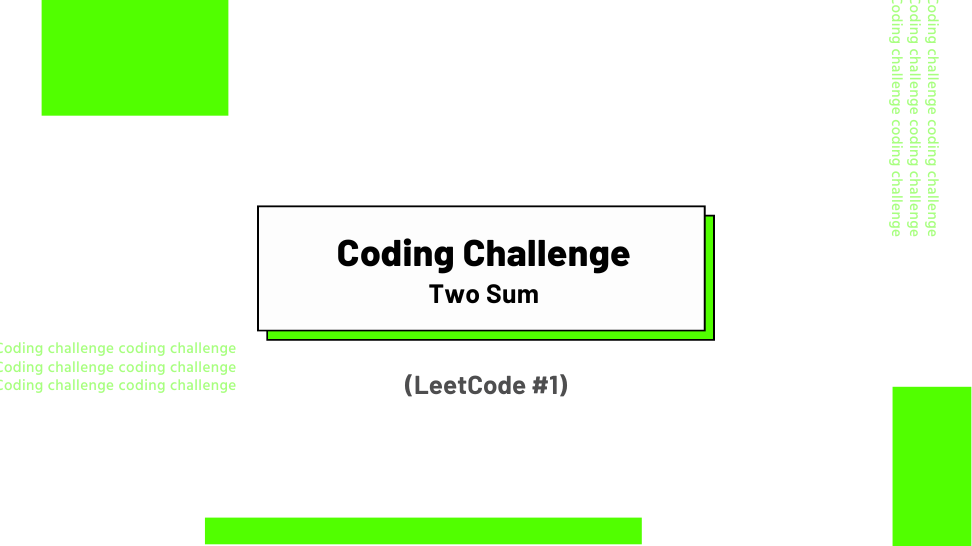## Challenge Statement

• Given an array of integers, nums, and an integer target, target, return indices of the two numbers such that they add up to target.
• You may assume that each input would have exactly one solution, and you may not use the same element twice.
• You can return the answer in any order.
• This challenge corresponds to LeetCode #1.

### Constraints

• `2 <= len(nums) <= 104`
• `-109 <= nums[i] <= 109`
• `-109 <= target <= 109`
• Only one valid answer exists.

### Example 1:

Input:` nums = [2, 7, 11, 15]`, `target = 9`

Output: `[0, 1]`

Explanation: Because `nums + nums == 9`, we return` [0, 1]`.

### Example 2:

Input: `nums = [3, 2, 4]`, `target = 6`

Output: `[1, 2]`

### Example 3:

Input:` nums = [3, 3]`, `target = 6`

Output: `[0, 1]`

## Solution

Below is my solution and some test cases. This solution has a linear time complexity O(n) and a linear space complexity O(n), where n is the length of the input list nums.